# The N-queens Problem

In the following sections, we'll illustrate constraint programming (CP) by a combinatorial problem based on the game of chess. In chess, a queen can attack horizontally, vertically, and diagonally. The N-queens problem asks:

How can N queens be placed on an NxN chessboard so that no two of them attack each other?

Below, you can see one possible solution to the N-queens problem for N = 4.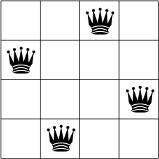No two queens are on the same row, column, or diagonal.

Note that this isn't an optimization problem: we want to find all possible solutions, rather than one optimal solution, which makes it a natural candidate for constraint programming. The following sections describe the CP approach to the N-queens problem, and present Python programs that solve it using both the CP-SAT solver and the original CP solver.

## CP approach to the N-queens problem

A CP solver works by systematically trying all possible assignments of values to the variables in a problem, to find the feasible solutions. In the 4-queens problem, the solver starts at the leftmost column and successively places one queen in each column, at a location that is not attacked by any previously placed queens.

### Propagation and backtracking

There are two key elements to a constraint programming search:

• Propagation — Each time the solver assigns a value to a variable, the constraints add restrictions on the possible values of the unassigned variables. These restrictions propagate to future variable assignments. For example, in the 4-queens problem, each time the solver places a queen, it can't place any other queens on the row and diagonals the current queen is on. Propagation can speed up the search significantly by reducing the set of variable values the solver must explore.
• Backtracking occurs when either the solver can't assign a value to the next variable, due to the constraints, or it finds a solution. In either case, the solver backtracks to a previous stage and changes the value of the variable at that stage to a value that hasn't already been tried. In the 4-queens example, this means moving a queen to a new square on the current column.

Next, you'll see how constraint programming uses propagation and backtracking to solve the 4-queens problem.

Let's suppose the solver starts by arbitrarily placing a queen in the upper left corner. That's a hypothesis of sorts; perhaps it will turn out that no solution exists with a queen in the upper left corner.

Given this hypothesis, what constraints can we propagate? One constraint is that there can be only one queen in a column (the gray Xs below), and another constraint prohibits two queens on the same diagonal (the red Xs below).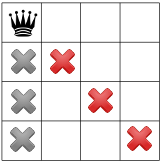Our third constraint prohibits queens on the same row: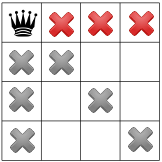Our constraints propagated, we can test out another hypothesis, and place a second queen on one of the available remaining squares. Our solver might decide to place in it the first available square in the second column: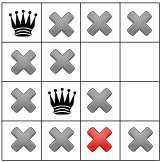After propagating the diagonal constraint, we can see that it leaves no available squares in either the third column or last row: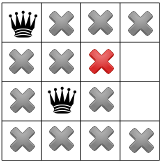With no solutions possible at this stage, we need to backtrack. One option is for the solver to choose the other available square in the second column. However, constraint propagation then forces a queen into the second row of the third column, leaving no valid spots for the fourth queen: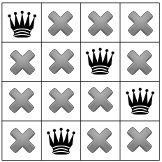And so the solver must backtrack again, this time all the way back to the placement of the first queen. We have now shown that no solution to the queens problem will occupy a corner square.

Since there can be no queen in the corner, the solver moves the first queen down by one, and propagates, leaving only one spot for the second queen: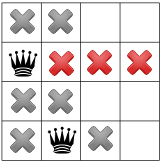Propagating again reveals only one spot left for the third queen: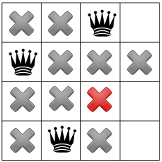And for the fourth and final queen: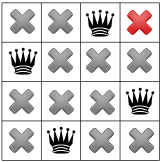We have our first solution! If we instructed our solver to stop after finding the first solution, it would end here. Otherwise, it would backtrack again and place the first queen in the third row of the first column.

## Solution using CP-SAT

The N-queens problem is ideally suited to constraint programming. In this section we'll walk through a short Python program that uses the CP-SAT solver to find all solutions to the problem.

### Declare the model

The following code declares the CP-SAT model.

```from ortools.sat.python import cp_model

def main(board_size):
model = cp_model.CpModel()```

### Create the variables

The solver's `NewIntVar` method creates the variables for the problem as an array named `queens`.

```  queens = [model.NewIntVar(0, board_size - 1, 'x%i' % i)
for i in range(board_size)]```

Here we assume that `queens[j]` is the row number for the queen in column `j`. In other words, `queens[j] = i` means there is a queen in row `i` and column `j`.

Note: When drawing a diagram of a solution, you will get a different picture depending on whether the rows are ordered from bottom to top or top to bottom (and whether the columns are ordered from left to right or vice versa). However, the ordering doesn't change the set of all possible solutions, just the way they are represented in a diagram.

### Create the constraints

Here's the code that creates the constraints for the problem.

```  # The following sets the constraint that all queens are in different rows.

# Note: all queens must be in different columns because the indices of queens are all different.

# The following sets the constraint that no two queens can be on the same diagonal.
for i in range(board_size):
# Note: is not used in the inner loop.
diag1 = []
diag2 = []
for j in range(board_size):
# Create variable array for queens(j) + j.
q1 = model.NewIntVar(0, 2 * board_size, 'diag1_%i' % i)
diag1.append(q1)
# Create variable array for queens(j) - j.
q2 = model.NewIntVar(-board_size, board_size, 'diag2_%i' % i)
diag2.append(q2)

The code uses the `AddAllDifferent` method, which requires all the elements of a variable array to be different.

Let's see how these constraints guarantee the three conditions for the N-queens problem (queens on different rows, columns, and diagonals).

#### No two queens on the same row

Applying the solver's `AllDifferent` method to `queens` forces the values of ``` queens[j]``` to be different for each `j`, which means that all queens must be in different rows.

#### No two queens on the same column

This constraint is implicit in the definition of `queens`. Since no two elements of `queens` can have the same index, no two queens can be in the same column.

#### No two queens on the same diagonal

The diagonal constraint is a little trickier than the row and column constraints. First, if two queens lie on the same diagonal, one of the following conditions must be true:

• The row number plus the column number for each of the two queens are equal. In other words, `queens(j) + j` has the same value for two different indices `j`.
• The row number minus the column number for each of the two queens are equal. In this case, `queens(j) - j` has the same value for two different indices `j`.

One of these conditions means the queens lie on the same ascending diagonal (going from left to right), while the other means they lie on the same descending diagonal. Which condition corresponds to ascending and which to descending depends on how you order the rows and columns. As mentioned in the previous section, the ordering has no effect on the set of solutions, just on how you visualize them.

So the diagonal constraint is that the values of `queens(j) + j` must all be different, and the values of `queens(j) - j` must all be different, for different `j`.

To apply the `AddAllDifferent` method to `queens(j) + j`, we put the N instances of the variable, for `j` from 0 to N - 1, into an array, `diag1`, as follows:

```q1 = model.NewIntVar(0, 2 * board_size, 'diag1_%i' % i)
diag1.append(q1)

Then we apply `AddAllDifferent` to `diag1`.

`model.AddAllDifferent(diag1)`

The constraint for `queens(j) - j` is created similarly.

### Create a solution printer

To print all solutions to the N-queens problem, you need to pass a callback, called a solution printer, to the CP-SAT solver. The callback prints each new solution as the solver finds it. The following code creates a solution printer.

```class SolutionPrinter(cp_model.CpSolverSolutionCallback):
"""Print intermediate solutions."""

def __init__(self, variables):
cp_model.CpSolverSolutionCallback.__init__(self)
self.__variables = variables
self.__solution_count = 0

def OnSolutionCallback(self):
self.__solution_count += 1
for v in self.__variables:
print('%s = %i' % (v, self.Value(v)), end = ' ')
print()

def SolutionCount(self):
return self.__solution_count```

Note that the solution printer must be written as a Python class, due to the Python interface to the underlying C++ solver.

The solutions are printed by the following lines in the solution printer.

```for v in self.__variables:
print('%s = %i' % (v, self.Value(v)), end = ' ')```

In this example, `self.__variables` is the variable `queens`, and each `v` corresponds to one of the eight entries of `queens`. This prints a solution in the following form:

`x0 = queens(0) x1 = queens(1) ... x7 = queens(7)` where `xi` is the column number of the queen in row `i`.

The next section shows an example of a solution.

### Call the solver and display the results

The following code runs the solver and displays the solutions.

```  solver = cp_model.CpSolver()
solution_printer = SolutionPrinter(queens)
status = solver.SearchForAllSolutions(model, solution_printer)
print()
print('Solutions found : %i' % solution_printer.SolutionCount())```

The program finds 92 different solutions for an 8x8 board. Here's the first one.

```x0 = 0 x1 = 6 x2 = 4 x3 = 7 x4 = 1 x5 = 3 x6 = 5 x7 = 2
...91 other solutions displayed...

Solutions found: 92```

The `xi` correspond to row numbers. So, for example, `x0 = 3` means that the row number for the queen in the first column is 3.

### An alternative solution printer

The following code creates an alternative solution printer, which prints the solutions as chessboard diagrams.

```class DiagramPrinter(cp_model.CpSolverSolutionCallback):
def __init__(self, variables):
cp_model.CpSolverSolutionCallback.__init__(self)
self.__variables = variables
self.__solution_count = 0

def OnSolutionCallback(self):
self.__solution_count += 1

for v in self.__variables:
queen_col = int(self.Value(v))
board_size = len(self.__variables)
# Print row with queen.
for j in range(board_size):
if j == queen_col:
# There is a queen in column j, row i.
print("Q", end=" ")
else:
print("_", end=" ")
print()
print()

def SolutionCount(self):
return self.__solution_count```

You create this solution printer by the call

`solution_printer = DiagramPrinter(queens)`

Now when you run the program, it displays the diagrams for all solutions. The diagram for the first solution is

```Q _ _ _ _ _ _ _
_ _ _ _ _ _ Q _
_ _ _ _ Q _ _ _
_ _ _ _ _ _ _ Q
_ Q _ _ _ _ _ _
_ _ _ Q _ _ _ _
_ _ _ _ _ Q _ _
_ _ Q _ _ _ _ _

...91 other solutions displayed...

Solutions found: 92```

You can solve the problem for a board of a different size by passing in N as a command-line argument. For example, if the program is named `queens`,

`python queens.py 6`

solves the problem for a 6x6 board.

### The entire program

Here is the entire program for the N-queens program.

```from __future__ import print_function
import sys
from ortools.sat.python import cp_model

def main(board_size):
model = cp_model.CpModel()
# Creates the variables.
# The array index is the column, and the value is the row.
queens = [model.NewIntVar(0, board_size - 1, 'x%i' % i)
for i in range(board_size)]
# Creates the constraints.
# The following sets the constraint that all queens are in different rows.

# Note: all queens must be in different columns because the indices of queens are all different.

# The following sets the constraint that no two queens can be on the same diagonal.
for i in range(board_size):
# Note: is not used in the inner loop.
diag1 = []
diag2 = []
for j in range(board_size):
# Create variable array for queens(j) + j.
q1 = model.NewIntVar(0, 2 * board_size, 'diag1_%i' % i)
diag1.append(q1)
# Create variable array for queens(j) - j.
q2 = model.NewIntVar(-board_size, board_size, 'diag2_%i' % i)
diag2.append(q2)
### Solve model.
solver = cp_model.CpSolver()
solution_printer = SolutionPrinter(queens)
status = solver.SearchForAllSolutions(model, solution_printer)
print()
print('Solutions found : %i' % solution_printer.SolutionCount())

class SolutionPrinter(cp_model.CpSolverSolutionCallback):
"""Print intermediate solutions."""

def __init__(self, variables):
cp_model.CpSolverSolutionCallback.__init__(self)
self.__variables = variables
self.__solution_count = 0

def OnSolutionCallback(self):
self.__solution_count += 1
for v in self.__variables:
print('%s = %i' % (v, self.Value(v)), end = ' ')
print()

def SolutionCount(self):
return self.__solution_count

class DiagramPrinter(cp_model.CpSolverSolutionCallback):
def __init__(self, variables):
cp_model.CpSolverSolutionCallback.__init__(self)
self.__variables = variables
self.__solution_count = 0

def OnSolutionCallback(self):
self.__solution_count += 1

for v in self.__variables:
queen_col = int(self.Value(v))
board_size = len(self.__variables)
# Print row with queen.
for j in range(board_size):
if j == queen_col:
# There is a queen in column j, row i.
print("Q", end=" ")
else:
print("_", end=" ")
print()
print()

def SolutionCount(self):
return self.__solution_count

if __name__ == '__main__':
# By default, solve the 8x8 problem.
board_size = 8
if len(sys.argv) > 1:
board_size = int(sys.argv)
main(board_size)
```

## Solution using the original CP solver

The following sections present a Python program that solves N-queens using the original CP solver. (However, we recommend using the newer CP-SAT solver.)

### Declare the solver

The following code declares the original CP solver.

```from ortools.constraint_solver import pywrapcp

def main(board_size):
# Creates the solver.
solver = pywrapcp.Solver("n-queens")```

### Create the variables

The solver's `IntVar` method creates the variables for the problem as an array named `queens`.

```  # Creates the variables.
# The array index is the column, and the value is the row.
queens = [solver.IntVar(0, board_size - 1, "x%i" % i) for i in range(board_size)]```

For any solution, `queens[j] = i` means there is a queen in column `j` and row `i`.

### Create the constraints

Here's the code that creates the constraints for the problem.

```  # Creates the constraints.

# All rows must be different.

# All columns must be different because the indices of queens are all different.

# No two queens can be on the same diagonal.
solver.Add(solver.AllDifferent([queens[i] + i for i in range(board_size)]))
solver.Add(solver.AllDifferent([queens[i] - i for i in range(board_size)]))
```

These constraints guarantee the three conditions for the N-queens problem (queens on different rows, columns, and diagonals).

#### No two queens on the same row

Applying the solver's `AllDifferent` method to `queens` forces the values of ``` queens[j]``` to be different for each `j`, which means that all queens must be in different rows.

#### No two queens on the same column

This constraint is implicit in the definition of `queens`. Since no two elements of `queens` can have the same index, no two queens can be in the same column.

#### No two queens on the same diagonal

The diagonal constraint is a little trickier than the row and column constraints. First, if two queens lie on the same diagonal, one of the following must be true:

• If the diagonal is descending (going from left to right), the row number plus column number for each of the two queens are equal. So `queens(i) + i` has the same value for two different indices `i`.
• If the diagonal is ascending, the row number minus the column number for each of the two queens are equal. In this case, `queens(i) - i` has the same value for two different indices `i`.

So the diagonal constraint is that the values of `queens(i) + i` must all be different, and likewise the values of `queens(i) - i` must all be different, for different `i`.

The code above adds this constraint by applying the `AllDifferent` method to `queens[j] + j` and `queens[j] - j`, for each `i`.

The next step is to create a decision builder, which sets the search strategy for the problem. The search strategy can have a major impact on the search time, due to propagation of constraints, which reduces the number of variable values the solver has to explore. You have already seen an example of this in the 4-queens example.

The following code creates a decision builder using the solver's `Phase` method.

```  db = solver.Phase(queens,
solver.CHOOSE_FIRST_UNBOUND,
solver.ASSIGN_MIN_VALUE)```
See Decision builder for details on the input arguments to the `Phase` method.

#### How the decision builder works in the 4-queens example

Let's take a look at how decision builder directs the search in the 4-queens example. The solver begins with `queens`, the first variable in the array, as directed by `CHOOSE_FIRST_UNBOUND`. The solver then assigns `queens` the smallest value that hasn't already been tried, which is 0 at this stage, as directed by `ASSIGN_MIN_VALUE`. This places the first queen in the upper left corner of the board.

Next, the solver selects `queens`, which is now the first unbound variable in `queens`. After propagating the constraints, there are two possible rows for a queen in column 1: row 2 or row 3. The `ASSIGN_MIN_VALUE` option directs the solver to assign `queens = 2`. (If instead, you set `IntValueStrategy` to `ASSIGN_MAX_VALUE`, the solver would assign `queens = 3`.)

You can check that the rest of the search follows the same rules.

### Call the solver and display the results

The following code runs the solver and displays the solution.

```  solver.NewSearch(db)

# Iterates through the solutions, displaying each.
num_solutions = 0

while solver.NextSolution():
# Displays the solution just computed.
for i in range(board_size):
for j in range(board_size):
if queens[j].Value() == i:
# There is a queen in column j, row i.
print("Q", end=" ")
else:
print("_", end=" ")
print()
print()
num_solutions += 1

solver.EndSearch()```

Here's the first solution found by the program for an 8x8 board.

```Q _ _ _ _ _ _ _
_ _ _ _ _ _ Q _
_ _ _ _ Q _ _ _
_ _ _ _ _ _ _ Q
_ Q _ _ _ _ _ _
_ _ _ Q _ _ _ _
_ _ _ _ _ Q _ _
_ _ Q _ _ _ _ _

...91 other solutions displayed...

Solutions found: 92```

You can solve the problem for a board of a different size by passing in N as a command-line argument. For example,

`python projects/queens.py 6`

solves the problem for a 6x6 board.

The number of solutions goes up roughly exponentially with the size of the board:

Board sizeSolutionsTime to find all solutions (ms)
110
200
300
420
5100
640
7403
8929
935235
1072495
112680378
12142002198
137371211628
1436559662427
152279184410701
Many solutions are just rotations of others, and a technique called symmetry breaking can be used to reduce the amount of computation needed. We don't use that here; our solution above isn't intended to be fast, just simple. Of course, we could make it much faster if we wanted to only find one solution instead of all of them: no more than a few milliseconds for board sizes up to 50.

### The entire program

The complete program is shown belos.

```"""
OR-tools solution to the N-queens problem.
"""
from __future__ import print_function
import sys
from ortools.constraint_solver import pywrapcp

def main(board_size):
# Creates the solver.
solver = pywrapcp.Solver("n-queens")
# Creates the variables.
# The array index is the column, and the value is the row.
queens = [solver.IntVar(0, board_size - 1, "x%i" % i) for i in range(board_size)]
# Creates the constraints.

# All rows must be different.

# All columns must be different because the indices of queens are all different.

# No two queens can be on the same diagonal.
solver.Add(solver.AllDifferent([queens[i] + i for i in range(board_size)]))
solver.Add(solver.AllDifferent([queens[i] - i for i in range(board_size)]))

db = solver.Phase(queens,
solver.CHOOSE_FIRST_UNBOUND,
solver.ASSIGN_MIN_VALUE)
solver.NewSearch(db)

# Iterates through the solutions, displaying each.
num_solutions = 0

while solver.NextSolution():
# Displays the solution just computed.
for i in range(board_size):
for j in range(board_size):
if queens[j].Value() == i:
# There is a queen in column j, row i.
print("Q", end=" ")
else:
print("_", end=" ")
print()
print()
num_solutions += 1

solver.EndSearch()

print()
print("Solutions found:", num_solutions)
print("Time:", solver.WallTime(), "ms")

# By default, solve the 8x8 problem.
board_size = 8

if __name__ == "__main__":
if len(sys.argv) > 1:
board_size = int(sys.argv)
main(board_size)```